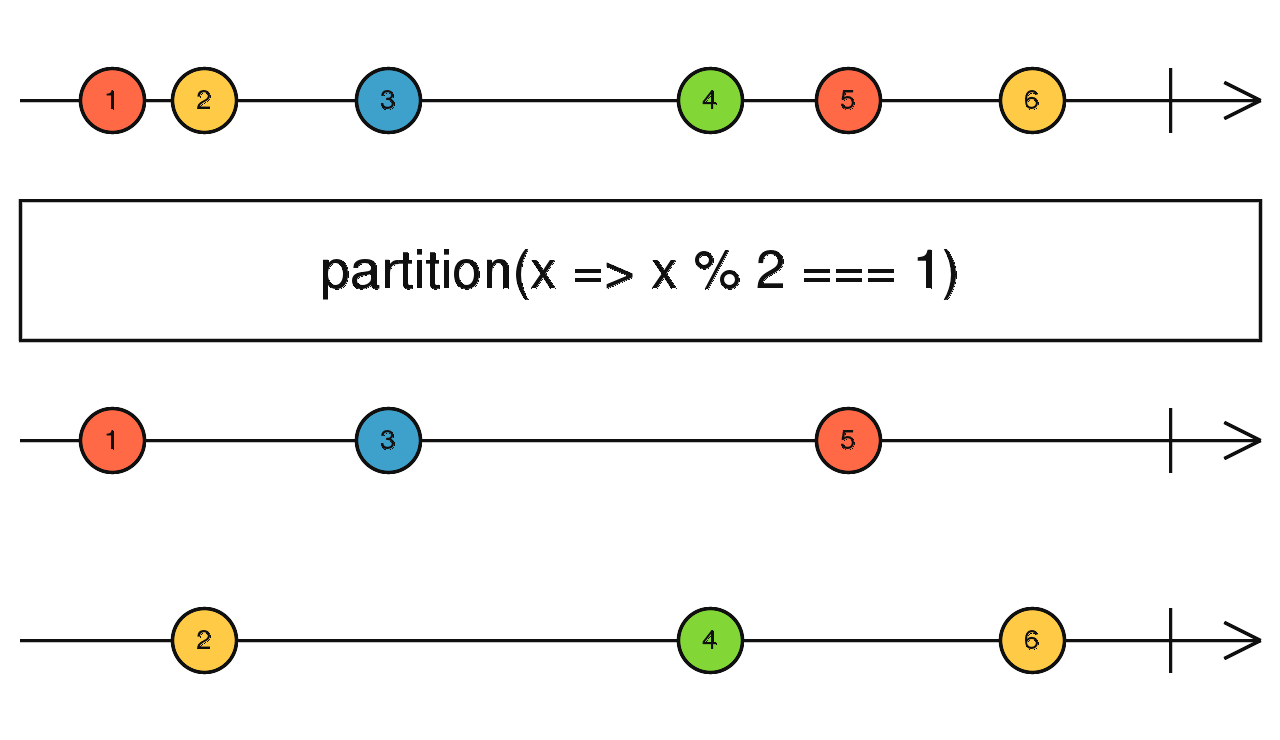/RxJS

# partition

`function` `stable`

Splits the source Observable into two, one with values that satisfy a predicate, and another with values that don't satisfy the predicate.

### `partition<T>(source: ObservableInput<T>, predicate: (value: T, index: number) => boolean, thisArg?: any): [Observable<T>, Observable<T>]`

#### Parameters

 source Type: `ObservableInput`. predicate A function that evaluates each value emitted by the source Observable. If it returns `true`, the value is emitted on the first Observable in the returned array, if `false` the value is emitted on the second Observable in the array. The `index` parameter is the number `i` for the i-th source emission that has happened since the subscription, starting from the number `0`. thisArg Optional. Default is `undefined`. An optional argument to determine the value of `this` in the `predicate` function.

#### Returns

`[Observable<T>, Observable<T>]`: An array with two Observables: one with values that passed the predicate, and another with values that did not pass the predicate.

## Description

It's like `filter`, but returns two Observables: one like the output of `filter`, and the other with values that did not pass the condition.`partition` outputs an array with two Observables that partition the values from the source Observable through the given `predicate` function. The first Observable in that array emits source values for which the predicate argument returns true. The second Observable emits source values for which the predicate returns false. The first behaves like `filter` and the second behaves like `filter` with the predicate negated.

## Example

Partition a set of numbers into odds and evens observables

```import { of, partition } from 'rxjs';

const observableValues = of(1, 2, 3, 4, 5, 6);
const [evens\$, odds\$] = partition(observableValues, (value, index) => value % 2 === 0);

odds\$.subscribe(x => console.log('odds', x));
evens\$.subscribe(x => console.log('evens', x));

// Logs:
// odds 1
// odds 3
// odds 5
// evens 2
// evens 4
// evens 6```